# RD Sharma Solutions for Class 9 Maths Chapter 3 Rationalisation Exercise VSAQs

RD Sharma Class 9 Mathematics Chapter 3 Exercise VSAQs Rationalisation PDF is available here. The subject matter experts have curated RD Sharma Class 9 Chapter 3 solutions as per the latest CBSE syllabus. Studying this chapter will help the students to score good marks in the final examination. Students can access RD Sharma Solutions for Class 9 Maths Chapter 3 Exercise VSAQs by clicking on the link below.

## Download PDF of RD Sharma Solutions for Class 9 Maths Chapter 3 Rationalisation Exercise VSAQs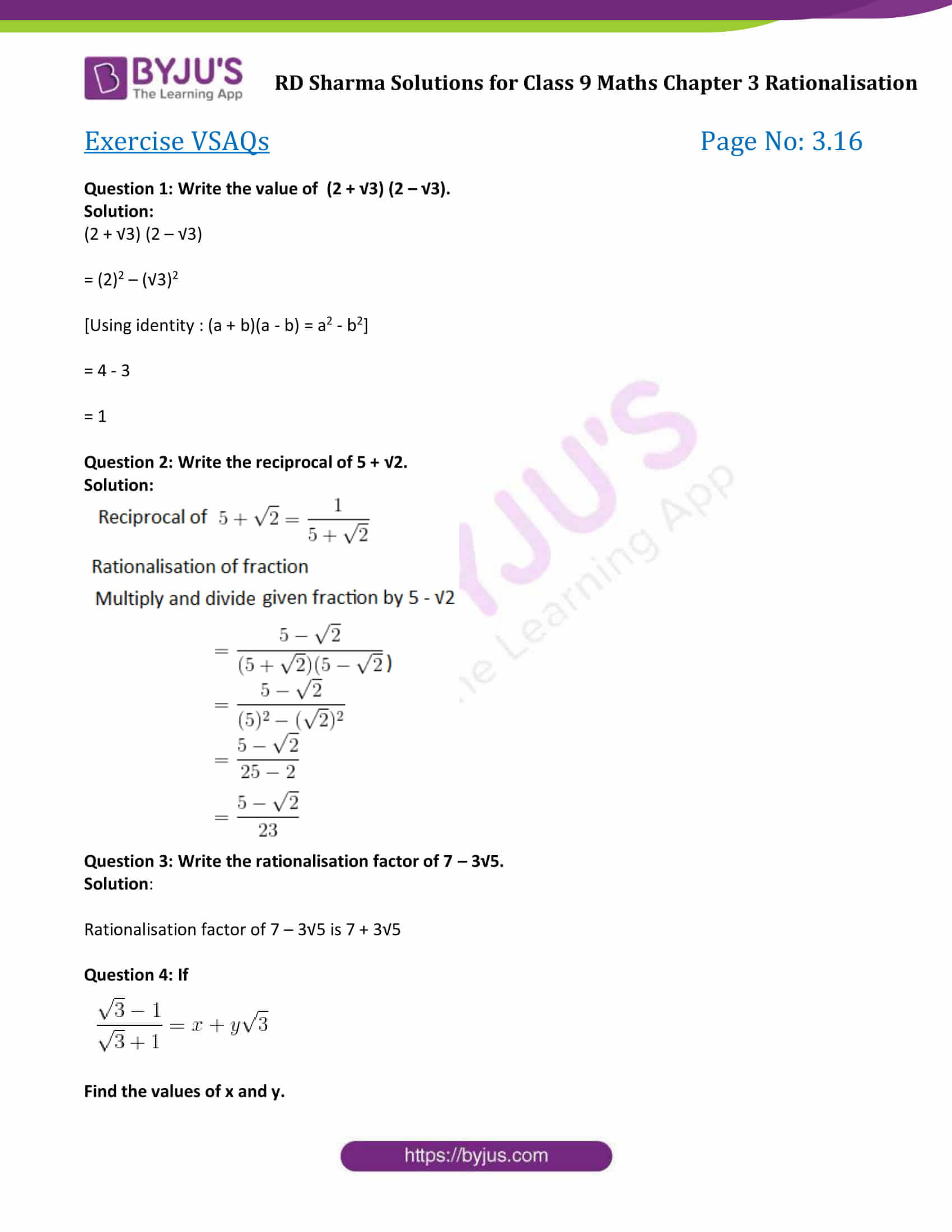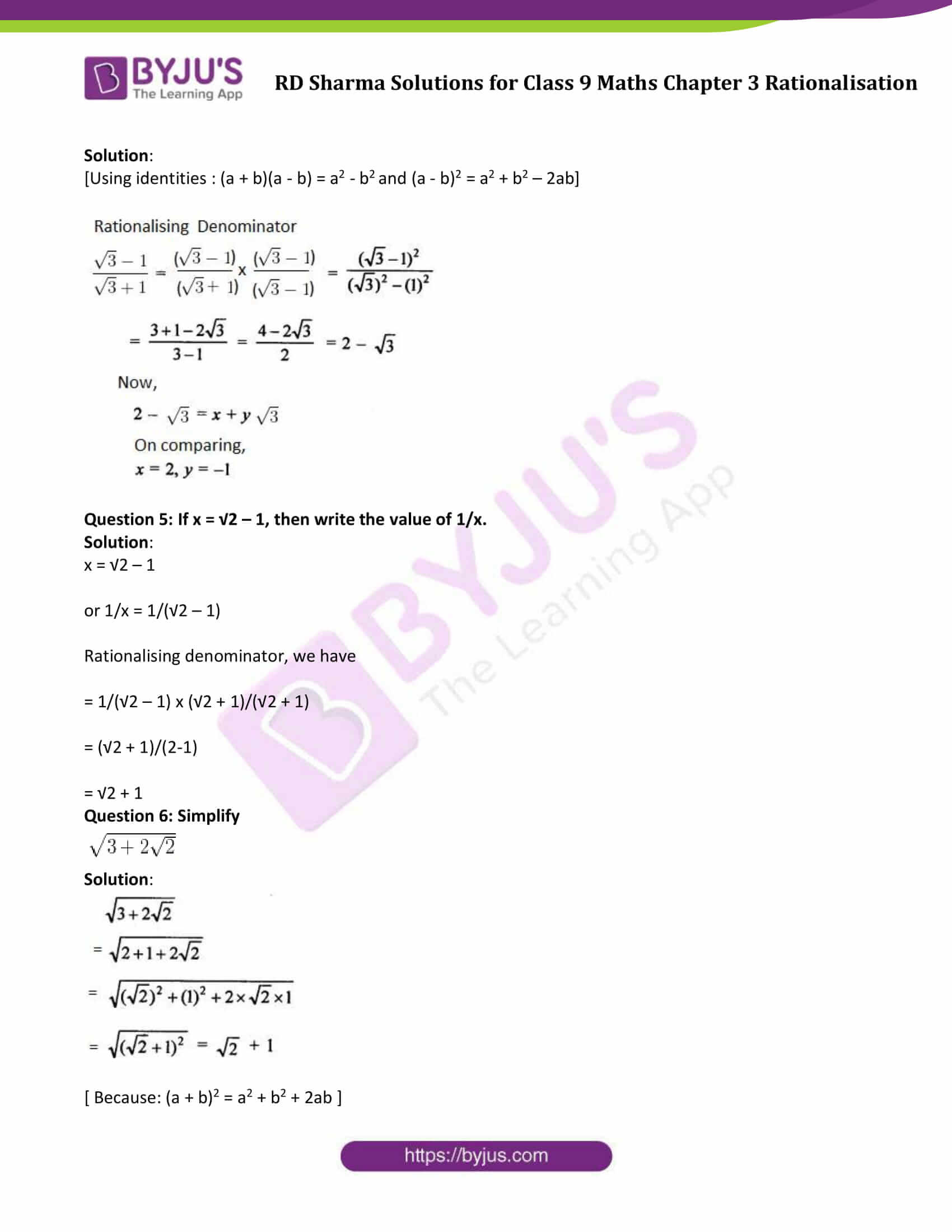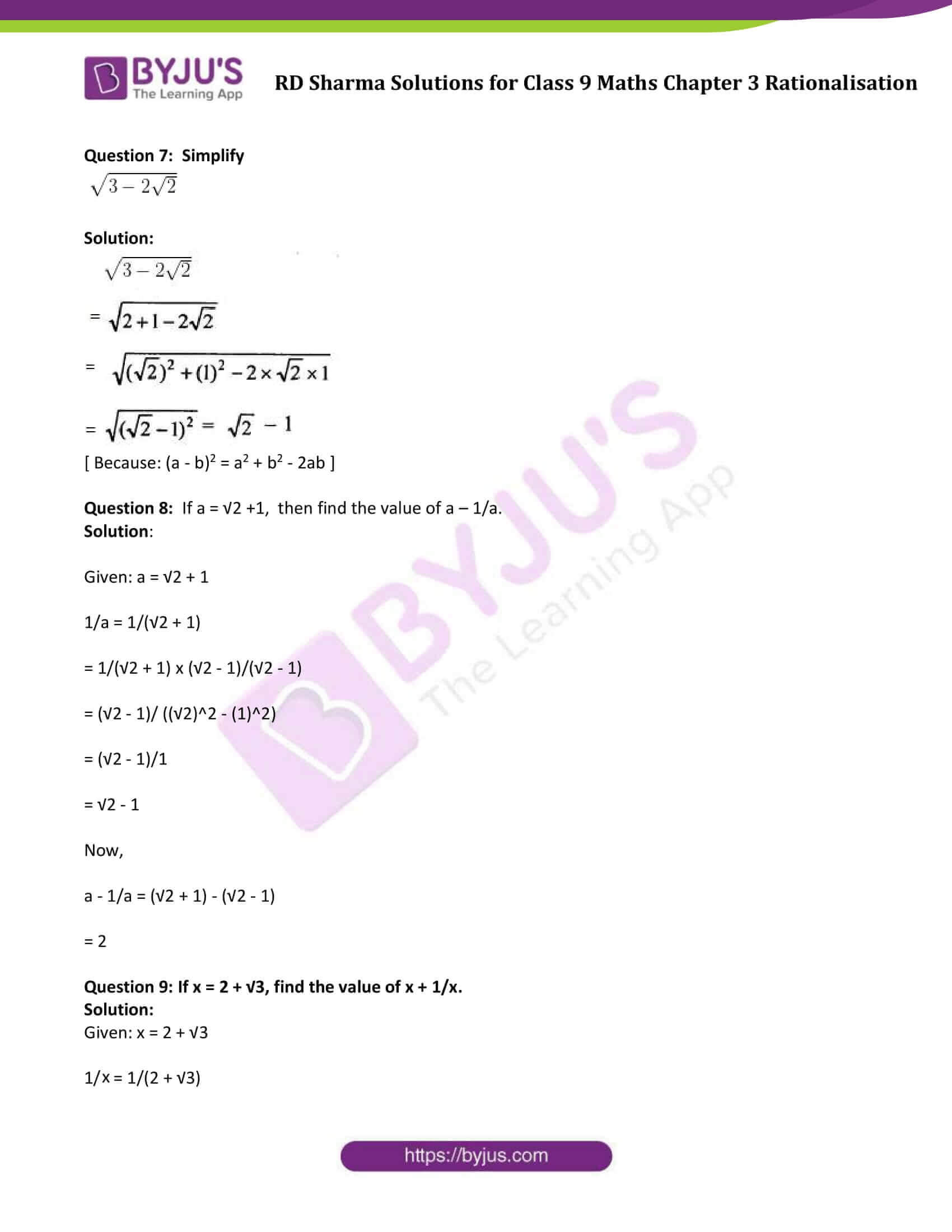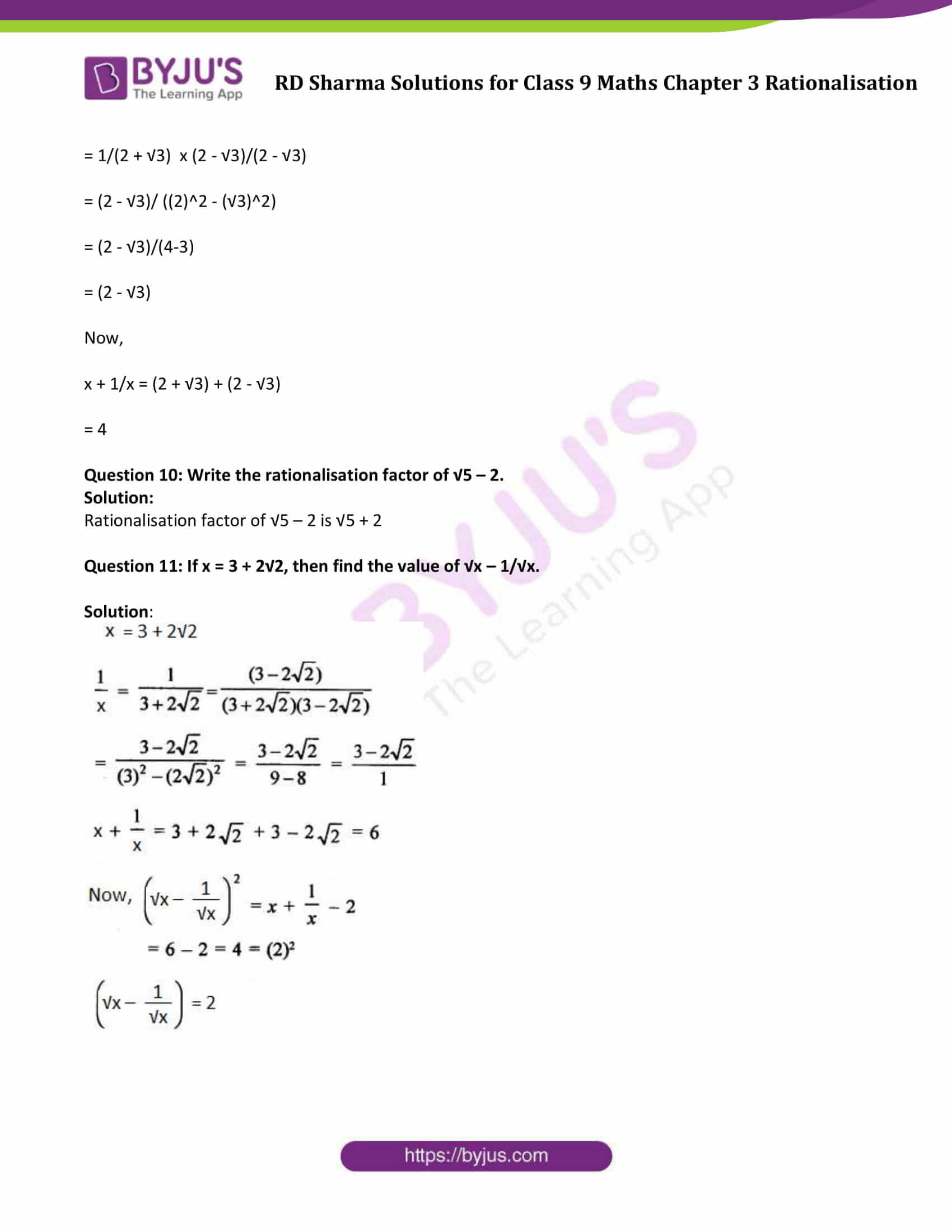### Access Answers to Maths RD Sharma Solutions for Class 9 Chapter 3 Rationalisation Exercise VSAQs

Question 1: Write the value of (2 + √3) (2 – √3).

Solution:

(2 + √3) (2 – √3)

= (2)2 – (√3)2

[Using identity : (a + b)(a – b) = a2 – b2]

= 4 – 3

= 1

Question 2: Write the reciprocal of 5 + √2.

Solution: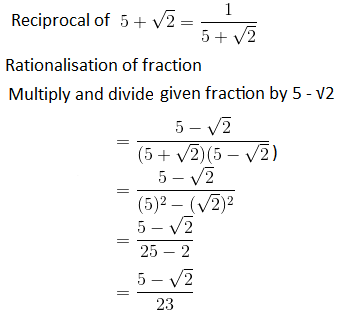Question 3: Write the rationalisation factor of 7 – 3√5.

Solution:

Rationalisation factor of 7 – 3√5 is 7 + 3√5

Question 4: If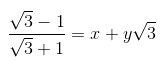Find the values of x and y.

Solution:

[Using identities : (a + b)(a – b) = a2 – b2 and (a – b)2 = a2 + b2 – 2ab]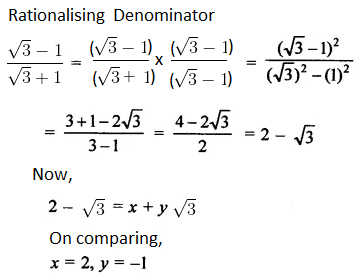Question 5: If x = √2 – 1, then write the value of 1/x.

Solution:

x = √2 – 1

or 1/x = 1/(√2 – 1)

Rationalising denominator, we have

= 1/(√2 – 1) x (√2 + 1)/(√2 + 1)

= (√2 + 1)/(2-1)

= √2 + 1

Question 6: Simplify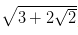Solution: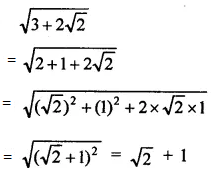[ Because: (a + b)2 = a2 + b2 + 2ab ]

Question 7: Simplify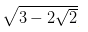Solution: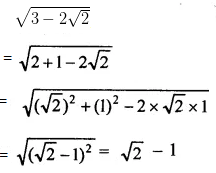[ Because: (a – b)2 = a2 + b2 – 2ab ]

Question 8: If a = √2 +1, then find the value of a – 1/a.

Solution:

Given: a = √2 + 1

1/a = 1/(√2 + 1)

= 1/(√2 + 1) x (√2 – 1)/(√2 – 1)

= (√2 – 1)/ ((√2)2 – (1)2)

= (√2 – 1)/1

= √2 – 1

Now,

a – 1/a = (√2 + 1) – (√2 – 1)

= 2

Question 9: If x = 2 + √3, find the value of x + 1/x.

Solution:

Given: x = 2 + √3

1/x = 1/(2 + √3)

= 1/(2 + √3) x (2 – √3)/(2 – √3)

= (2 – √3)/ ((2)2 – (√3)2)

= (2 – √3)/(4-3)

= (2 – √3)

Now,

x + 1/x = (2 + √3) + (2 – √3)

= 4

Question 10: Write the rationalisation factor of √5 – 2.

Solution:

Rationalisation factor of √5 – 2 is √5 + 2

Question 11: If x = 3 + 2√2, then find the value of √x – 1/√x.

Solution: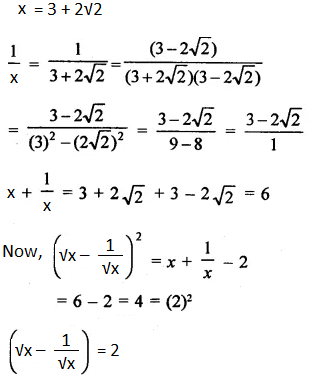## RD Sharma Solutions for Class 9 Maths Chapter 3 Rationalisation Exercise VSAQs

RD Sharma Solutions Class 9 Maths Chapter 3 Rationalisation Exercise VSAQs is based on the following topics:

• Use of some important algebraic identities
• Rationalisation of denominator